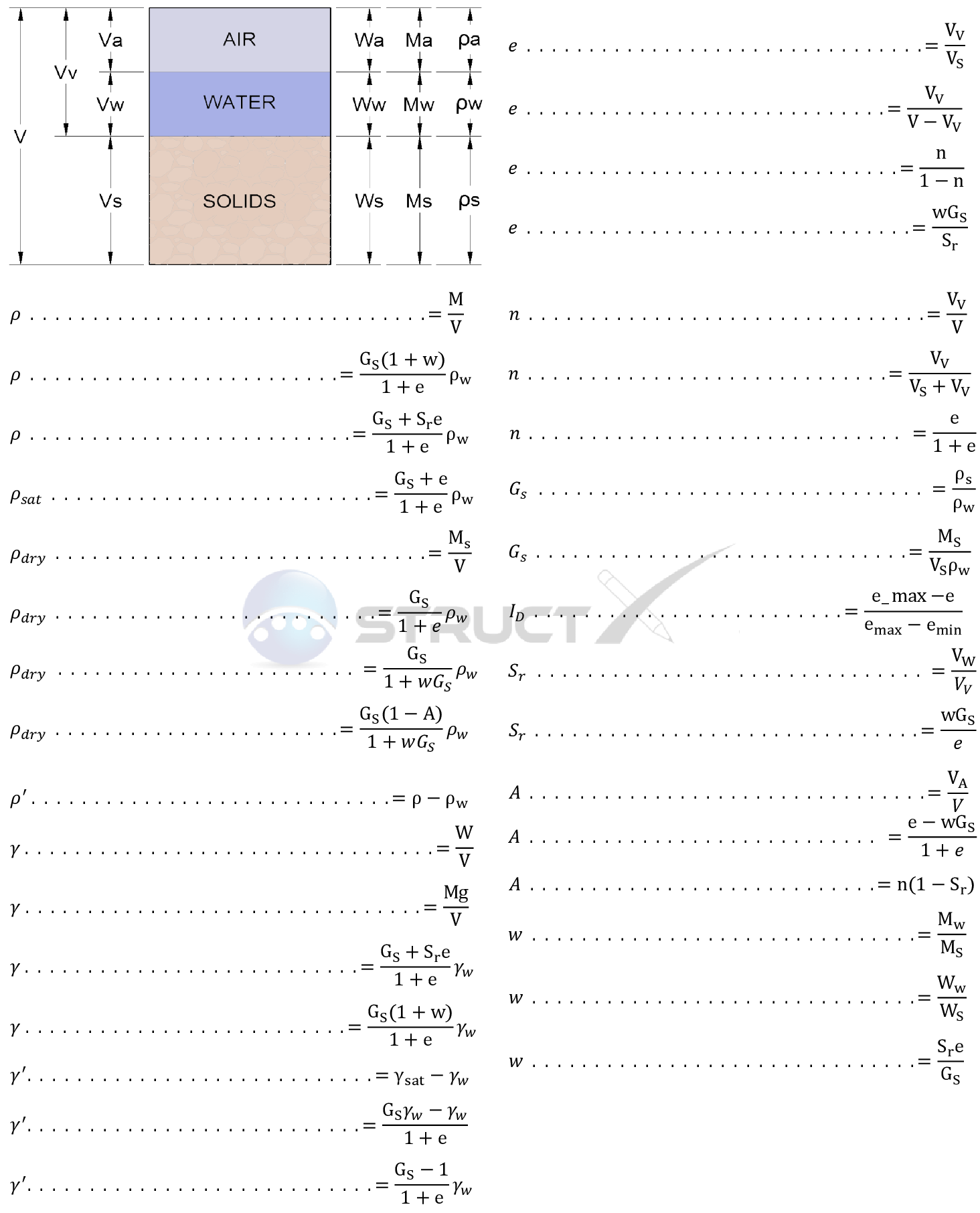# Soil Phase Diagram and Relationship FormulasMore Properties# Notation and Units

## Metric and Imperial Units

The above values have been provided with both imperial and metric units. As with all calculations care must be taken to keep consistent units throughout. Examples of suitable units have been shown below.

# Notation

• A = Air Content, percentage (%)
• e = void ratio (ratio of volume of voids to the volume of solids), no units
• Gs = specific gravity (the ratio of the density of the soil to the density of water), no units
• ID = density index (relative density), percentage (%)
• n = porosity (ratio of the volume of voids to the total volume), percentage (%)
• Sr = degree of saturation, percentage (%)
• w = water content, percentage (%)
• ρ = bulk density (the ratio of the total mass to the total volume), ib/ft3 or kg/m3
• ρsat = saturated bulk density (the ratio of the total saturated mass to the total volume), ib/ft3 or kg/m3
• ρdry = dry bulk density (the ratio of the total dry mass to the total volume), ib/ft3 or kg/m3
• ρ' = effective density (bulk density - density of water), ib/ft3 or kg/m3
• γ = unit weight(ratio of the total weight (force) to the total volume), ksi or KPa
• γ' = buoyant unit weight or effective unit weight(unit weight - unit weight of water ), ksi or KPa